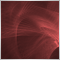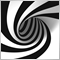# Unwanted reset of CCanvas position1136

Hello,

i need a canvas in my indicator. The canvas should be pegged to current close.
For this, i change it's position in OnCalculate.

The problem: When i switch between charts, very often the canvas position is somehow reset to the mid of chart
and will only be updated with the next tick.

I hardly dare to say it, but this behavior is unexpected. Why doesn't the canvas simply stay on its last position or updates correctly in the background (in OnCalculate call) ?

How could i fix this ?

The code:

```//+------------------------------------------------------------------+
//|                                                     cnv_test.mq5 |
//|                                                                  |
//|                                                                  |
//+------------------------------------------------------------------+
#property version   "1.00"
#property indicator_chart_window

#include <canvas/canvas.mqh>
CCanvas* _cv=NULL;
const int __x=100;
const int __y=100;
const int __w=400;
const int __h=400;
const string __name="my_canvas";
const int    __chart_id=0;
//+------------------------------------------------------------------+
//| Custom indicator initialization function                         |
//+------------------------------------------------------------------+
int OnInit()
{
_cv=new CCanvas;
ResetLastError();
bool bret=_cv.CreateBitmapLabel(__name,__x,__y,__w,__h,COLOR_FORMAT_XRGB_NOALPHA);
if(bret == false)
{
PrintFormat("%s %d",__FUNCTION__,GetLastError());
}
else
{
}
_cv.FillRectangle(__x,__y,__w,__h,clrWhite);

ObjectSetInteger(__chart_id,__name,OBJPROP_SELECTABLE,1);
ObjectSetInteger(__chart_id,__name,OBJPROP_SELECTED,1);

return(INIT_SUCCEEDED);
}
void OnDeinit(const int reason)
{
delete _cv;
}
//+------------------------------------------------------------------+
//| Custom indicator iteration function                              |
//+------------------------------------------------------------------+
int OnCalculate(const int rates_total,
const int prev_calculated,
const datetime &time[],
const double &open[],
const double &high[],
const double &low[],
const double &close[],
const long &tick_volume[],
const long &volume[],
{
//---
if(rates_total > 0)
{
double close=close[rates_total-1];
datetime dt=time[rates_total-1];

ResetLastError();
int new_x=0;
int new_y=0;
if(ChartTimePriceToXY(__chart_id,0,dt,close,new_x,new_y) == true)
{
ObjectSetInteger(__chart_id,__name,OBJPROP_XDISTANCE,new_x) ;
ObjectSetInteger(__chart_id,__name,OBJPROP_YDISTANCE,new_y) ;
}
else
PrintFormat("%s %d",__FUNCTION__,GetLastError());
}
//--- return value of prev_calculated for next call
return(rates_total);

}
//+------------------------------------------------------------------+
//| ChartEvent function                                              |
//+------------------------------------------------------------------+
void OnChartEvent(const int id,
const long &lparam,
const double &dparam,
const string &sparam)
{
//---

}
//+------------------------------------------------------------------+
```

Thank you6210

Hello,

i need a canvas in my indicator. The canvas should be pegged to current close.
For this, i change it's position in OnCalculate.

The problem: When i switch between charts, very often the canvas position is somehow reset to the mid of chart
and will only be updated with the next tick.

I hardly dare to say it, but this behavior is unexpected. Why doesn't the canvas simply stay on its last position or updates correctly in the background (in OnCalculate call) ?

How could i fix this ?

The code:

Thank you

when you change the chart symbol or timeframe your indicator is closed and restarted from scratch. If you want to avoid the outcome then you must change your code to reset the position accordingly on start up1136

Paul Anscombe:

when you change the chart symbol or timeframe your indicator is closed and restarted from scratch. If you want to avoid the outcome then you must change your code to reset the position accordingly on start up

Hello,

i neither change the symbol nor the timeframe.

Let's say, i have 3 charts open:

EURUSD, USDJPY, EURAUD. The chart window is maximized. So i just see 1 chart at once.

I attach my canvas indicator to EURUSD. With first tick, it is poisitioned to current close.

So far so good.

Now i switch to next chart USDJPY, want to see what's going on there.

Then i switch back to EURUSD and then my canvas is moved far to the left of the chart and not on last position or actual close position, which should be the case.1136

Short .mp4 video demonstrating this problem.
Files:
jumping_canvas.zip  3855 kb7488

Short .mp4 video demonstrating this problem.

The appearance of a square in the center is an MT5 bug.

But in order for the update to occur without waiting for a new tick, you need to add Update ():

```#property copyright ""
#property version   "1.00"
#property indicator_chart_window

#include <canvas/canvas.mqh>
CCanvas* _cv=new CCanvas;
const int __x=100;
const int __y=100;
const int __w=400;
const int __h=400;
const string __name="my_canvas";
const int    __chart_id=0;
double cur_pr = 0;
datetime cut_time = 0;
//+------------------------------------------------------------------+
//| Custom indicator initialization function                         |
//+------------------------------------------------------------------+
int OnInit() {
ResetLastError();
bool bret=_cv.CreateBitmapLabel(__name,__x,__y,__w,__h,COLOR_FORMAT_XRGB_NOALPHA);
if(bret == false) {
PrintFormat("%s %d",__FUNCTION__,GetLastError());
} else {
}
_cv.FillRectangle(__x,__y,__w,__h,clrWhite);
ObjectSetInteger(__chart_id,__name,OBJPROP_SELECTABLE,1);
ObjectSetInteger(__chart_id,__name,OBJPROP_SELECTED,1);
_cv.Update();
return(INIT_SUCCEEDED);
}
//+------------------------------------------------------------------+
//|                                                                  |
//+------------------------------------------------------------------+
void OnDeinit(const int reason) {
delete _cv;
}
//+------------------------------------------------------------------+
//| Custom indicator iteration function                              |
//+------------------------------------------------------------------+
int OnCalculate(const int rates_total,
const int prev_calculated,
const datetime &time[],
const double &open[],
const double &high[],
const double &low[],
const double &close[],
const long &tick_volume[],
const long &volume[],
//---
if(prev_calculated > 0) {
cur_pr = close[rates_total-1];
cut_time = time[rates_total-1];
ReDrawCanvas(cut_time,cur_pr);
}
//--- return value of prev_calculated for next call
return(rates_total);
}
//+------------------------------------------------------------------+
//| ChartEvent function                                              |
//+------------------------------------------------------------------+
void OnChartEvent(const int id,
const long &lparam,
const double &dparam,
const string &sparam) {
if (id == CHARTEVENT_CHART_CHANGE) ReDrawCanvas(cut_time,cur_pr);
}
//+------------------------------------------------------------------+
void ReDrawCanvas(datetime dt, double pr) {
ResetLastError();
int new_x=0;
int new_y=0;
if(ChartTimePriceToXY(__chart_id,0,dt,pr,new_x,new_y) == true) {
ObjectSetInteger(__chart_id,__name,OBJPROP_XDISTANCE,new_x) ;
ObjectSetInteger(__chart_id,__name,OBJPROP_YDISTANCE,new_y) ;
} else
PrintFormat("%s %d",__FUNCTION__,GetLastError());
_cv.Update();
}
//+------------------------------------------------------------------+
```7488

Short .mp4 video demonstrating this problem.

I've found the solution:

```#property copyright ""
#property version   "1.00"
#property indicator_chart_window

#include <canvas/canvas.mqh>
CCanvas* _cv=new CCanvas;
const int __x=100;
const int __y=100;
const int __w=400;
const int __h=400;
const string __name="my_canvas";
const int    __chart_id=0;
double cur_pr = 0;
datetime cut_time = 0;
//+------------------------------------------------------------------+
//| Custom indicator initialization function                         |
//+------------------------------------------------------------------+
int OnInit() {
ResetLastError();
bool bret=_cv.CreateBitmapLabel(__name,__x,__y,__w,__h,COLOR_FORMAT_XRGB_NOALPHA);
if(bret == false) {
PrintFormat("%s %d",__FUNCTION__,GetLastError());
} else {
}
_cv.FillRectangle(__x,__y,__w,__h,clrWhite);
ObjectSetInteger(__chart_id,__name,OBJPROP_SELECTABLE,1);
ObjectSetInteger(__chart_id,__name,OBJPROP_SELECTED,1);
_cv.Update();
return(INIT_SUCCEEDED);
}
//+------------------------------------------------------------------+
//|                                                                  |
//+------------------------------------------------------------------+
void OnDeinit(const int reason) {
delete _cv;
}
//+------------------------------------------------------------------+
//| Custom indicator iteration function                              |
//+------------------------------------------------------------------+
int OnCalculate(const int rates_total,
const int prev_calculated,
const datetime &time[],
const double &open[],
const double &high[],
const double &low[],
const double &close[],
const long &tick_volume[],
const long &volume[],
//---
if(prev_calculated > 0) {
cur_pr = close[rates_total-1];
cut_time = time[rates_total-1];
ReDrawCanvas(cut_time,cur_pr);
}
//--- return value of prev_calculated for next call
return(rates_total);
}
//+------------------------------------------------------------------+
//| ChartEvent function                                              |
//+------------------------------------------------------------------+
void OnChartEvent(const int id,
const long &lparam,
const double &dparam,
const string &sparam) {
if (id == CHARTEVENT_CHART_CHANGE) ReDrawCanvas(cut_time,cur_pr);
}
//+------------------------------------------------------------------+
void ReDrawCanvas(datetime dt, double pr) {
if (ChartGetInteger(0,CHART_BRING_TO_TOP)==0) return;
ResetLastError();
int new_x=0;
int new_y=0;
if(ChartTimePriceToXY(__chart_id,0,dt,pr,new_x,new_y) == true) {
ObjectSetInteger(__chart_id,__name,OBJPROP_XDISTANCE,new_x) ;
ObjectSetInteger(__chart_id,__name,OBJPROP_YDISTANCE,new_y) ;
} else
PrintFormat("%s %d",__FUNCTION__,GetLastError());
//_cv.Update();
}
//+------------------------------------------------------------------+```1136

Nikolai Semko:

I've found the solution:

Hello Nikolai,

many, many thanks. In fact, this removes the jumping and makes my indicator much more smooth.

One point, which is not related to this, just for asking.

It seems that

`CHARTEVENT_CHART_CHANGE`

is triggered when you change scale AND you switch from/to chart window. No way to distinguish between events.
If you are in contact with developers, could mention this. Perhaps it is possible to provide some additional information in the parameters ?

In any case, thank you!7488

Hello Nikolai,

many, many thanks. In fact, this removes the jumping and makes my indicator much more smooth.

One point, which is not related to this, just for asking.

It seems that

is triggered when you change scale AND you switch from/to chart window. No way to distinguish between events.
If you are in contact with developers, could mention this. Perhaps it is possible to provide some additional information in the parameters ?

In any case, thank you!

I agree that separation of event CHARTEVENT_CHART_CHANGE is needed.
I have already requested this several times.
But....silence.....